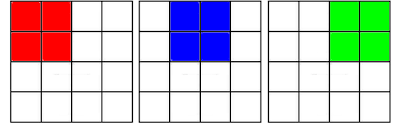## Sunday, August 26, 2012

### How many squares?

I keep seeing this puzzle on Facebook, so I'm posting my systematic solution here.There's a pattern of 16 basic squares in a regular 4  x  4 grid, plus two more superimposed on that grid to create 8 small squares.

First consider the basic squares.  There are 4 x 4 = 16 of them.

Now group them into 2 x 2 squares. There are 3 of those across:and there are obviously 3 of them down also, so there are 3 x 3 = 9 of them.

If you do the same using 3 x 3 squares, there are 2 across and 2 down, so there are 2 x 2 = 4 of them.

And of course if you group them into a 4 x 4 square, there's just 1 of those.

Now if you do the same with the two superimposed squares, there are 8 small squares and 2 large squares:So the total number of squares is:

16 + 9 + 4 + 1 + 8 + 2 = 40